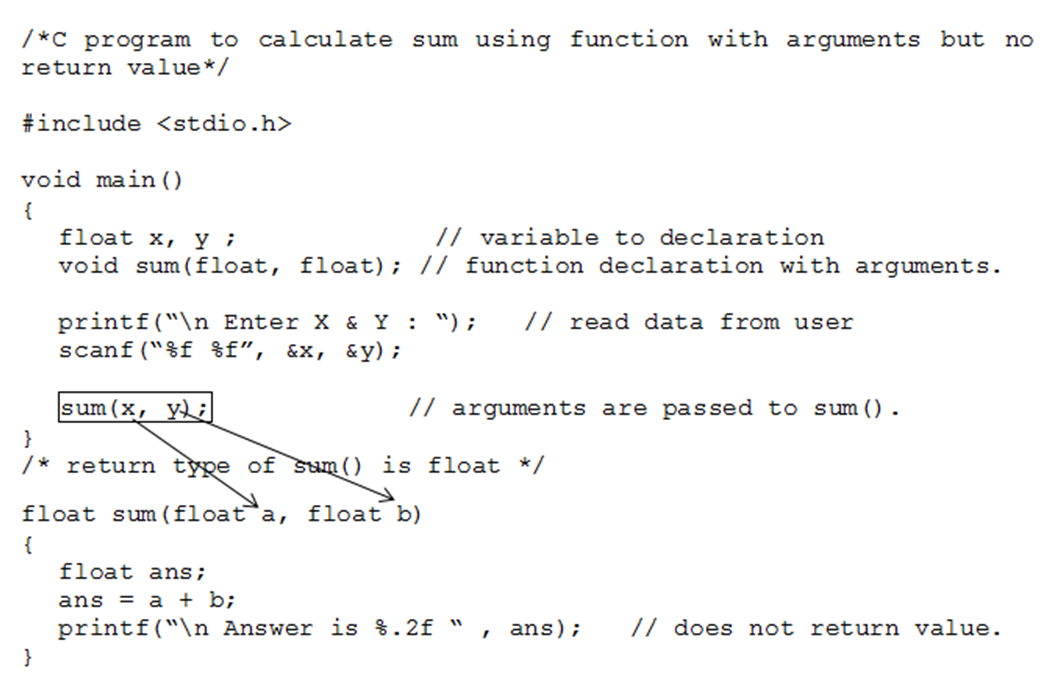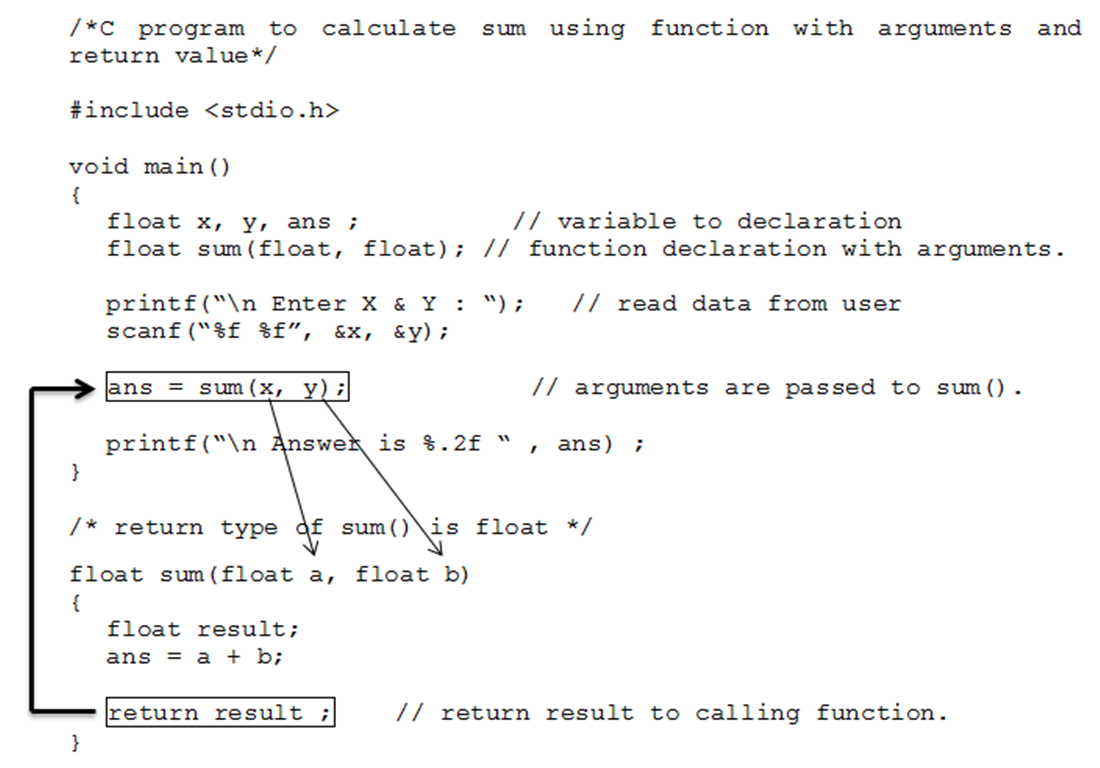• If you remember, we were talking about functions since last two posts. My previous post was on “Elements of Function”.
• In this post we will discuss about “Types or Categories of Function”.
• We can categorize functions into the following types based on function arguments and return value.
1. Function with no arguments and no return value
2. Function with no arguments and return value
3. Function with arguments but no return value
4. Function with arguments and return value.
5. Function that returns multiple values.

1. ### Function with no arguments and no return value

• This type of function neither receives any arguments nor returns any value.
• We need not to pass arguments while calling that function and we also don’t need any receiving parameter to receive return value as it does not return any value.
• The below example to find prime number implements function with no arguments and no return value.
```/*C program to check whether a number entered by user is prime or not using function with no arguments and no return value*/
#include "stdio.h"

void main()
{
void prime();        // function declaration
prime();            // No argument is passed to prime().
}
/* There is no return value to calling function main(). So, return type of prime() is void */

void prime()
{
int num,i,flag=0;
printf("Enter positive integer enter to check:\n");
scanf("%d",&num);
for(i=2;i<=num/2;++i)
{
if(num % i == 0)
{
flag = 1;
}
}
if (flag == 1)
printf("%d is not prime",num);
else
printf("%d is prime",num);
}
```
1. ### Function with no arguments and return value

• A function that does not take any argument but has return value falls under this category.
• Since it does not take any arguments, we need not to pass arguments during the function call but we need receiving variable to receive the value returned by the function.
• The following program to calculate sum implements function with no arguments and return value.
``````/*C program to calculate sum using function with no arguments and return value*/
#include "stdio.h"

void main()
{
float result ;          // variable to receive sum
float sum();            // function declaration
result = sum();        // No argument is passed to sum().
printf(“\n Sum is %.2f “ , result) ;
}
/* return type of sum() is float */

float sum()
{
float x, y, ans;
printf("Enter X & Y:\n");
scanf("%f %f",&x, &y);

ans = x + y;
return ans;    // returns result to calling function i.e. main()
}
``````
1. ### Function with arguments but no return value

• A function that takes arguments but does not return any value falls under this category.
• Since it takes arguments, we need to pass them during the function call but we don’t need receiving variable to receive the value as it does not return any value.
• The following program to calculate sum implements function with arguments and no return value.• Note that since function sum () does not have return type, we cannot use value calculated by sum () into main () so if we require further processing on calculated result inside main () then we have to provide return value and if no further processing is required inside the main () then its ok to use function with no return value.
1. ### Function with arguments and return value

• A function that takes arguments as well as return value falls under this category.
• Since it takes arguments, we need to pass them during the function call.
• We also need receiving variable to receive the value as it returns value.
• The following program to calculate sum implements function with arguments and return value.1. ### Function that returns multiple value

• Normally a function returns only single value but a function can also returns multiple values.
• We can make a function to return multiple values using pointer.
• The following program to swap values implements function that returns multiple value.
```/*C program to swap values using function with multiple return value by pointer*/

#include "stdio.h"

void main()
{
float x, y ;          // variable to declaration

void swap(float *, float *); //function declaration with pointer

printf(“\n Enter X & Y : “);   // read data from user
scanf(“%f %f”, &x, &y);

swap(&x, &y);       // arguments are passed by reference.

printf(“\n X & Y after swapping is %.2f %.2f “ , x, y);
}

/* return type of sum() is float */

float sum(float *a, float *b)
{
float temp ;

temp = *a ;       // swap value using pointer
*a = *b ;
*b = temp ;
}
```

Note: We will look into more detail about functions with pointer when we deal with pointers.